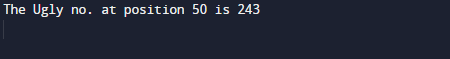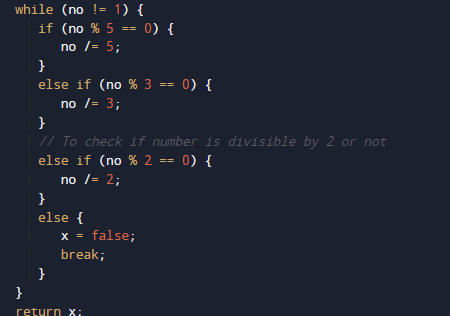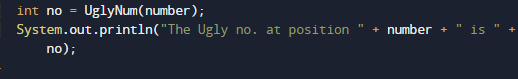# Program to check Ugly Number in Java

In this tutorial you will learn about the Program to check Ugly Number in Java and its application with practical example.

In this tutorial, we will learn to create a Java Program to check Ugly Number in Java using Java programming.

• What is Ugly Numbers?
• ## Prerequisites

Before starting with this tutorial we assume that you are best aware of the following Java programming topics:

• Java Operators.
• Basic Input and Output function in Java.
• Class and Object in Java.
• Basic Java programming.
• If-else statements in Java.
• For loop in Java.
• User define functions.

# What is Ugly Numbers?

Ugly numbers is a number whose prime factors are “2, 3 or 5“. Some of the Ugly number are as  “1, 2, 3, 4, 5, 6, 8, 9, 10, 12, 15”. So the number after dividing gives factors either 2,3 or 5, are called Ugly number.

## Program to check Ugly Number in Java

In this program , we will learn to create a Program to check Ugly Number.First we would declared and initialized the required variables. Next, we would prompt user to input the values. Later we will find that they the number is Ugly or not.

## outputIn the above program, we have first declared and initialized a set variables required in the program.

• number= A number N to find the Nth Ugly Number.
• no2= it will hold second entered number.
• sum1=  it will calculate first divisor’s sum.
• sum2=  it will calculate second divisor’s sum.
• i = for iteration.

After that taking  values from user we will assigned these values in to variable “no1” and “no2” respectively.

So what we are do here is that if any number has factor 2,3, or 5 if it has then the number is ugly and if number not have factors 2,3 or 5 then it is not ugly.Let’s take an example for that
Number is : 10
So Prime Factors of 10 is : 2 and 5.
Prime Factors contains only  2,3 or 5 : Here 10 is an Ugly number.

Number is : 11
So Prime Factors of 11 is : 1 and 11.
Prime Factors contains only  2,3 or 5 : Here 11 is not an Ugly number.

so here we have done same using java programming in image  below .So finding that the number is Ugly or not, we divide the number by  2, 3, and 5. When the number gives remainder equivalent to  Zero,then it is an Ugly number. Otherwise, it is not an Ugly number.In this tutorial we have learn about the Program to check Ugly Number in Java and its application with practical example. I hope you will like this tutorial.